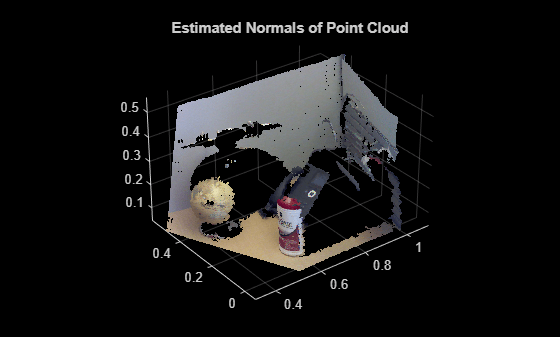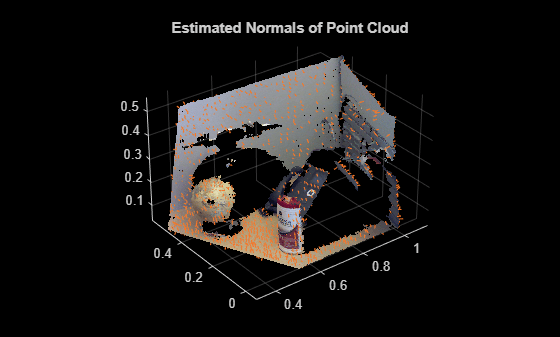# pcnormals

Estimate normals for point cloud

## Syntax

``normals = pcnormals(ptCloud)``
``normals = pcnormals(ptCloud,k)``

## Description

example

````normals = pcnormals(ptCloud)` returns a matrix that stores a normal for each point in the input `ptCloud`. The function uses six neighboring points to fit a local plane to determine each normal vector.```
``` `normals = pcnormals(ptCloud,k)` additionally specifies `k`, the number of points used for local plane fitting. The function uses this value rather than the six neighboring points as described in the first syntax.```

## Examples

collapse all

`load('object3d.mat');`

Estimate the normal vectors.

```normals = pcnormals(ptCloud); figure pcshow(ptCloud) title('Estimated Normals of Point Cloud') hold on``````x = ptCloud.Location(1:10:end,1:10:end,1); y = ptCloud.Location(1:10:end,1:10:end,2); z = ptCloud.Location(1:10:end,1:10:end,3); u = normals(1:10:end,1:10:end,1); v = normals(1:10:end,1:10:end,2); w = normals(1:10:end,1:10:end,3);```

Plot the normal vectors.

```quiver3(x,y,z,u,v,w); hold off```Flip the normals to point towards the sensor location. This step is necessary only for determining the inward or outward direction of the surface. The sensor center is set in x , y , z coordinates.

```sensorCenter = [0,-0.3,0.3]; for k = 1 : numel(x) p1 = sensorCenter - [x(k),y(k),z(k)]; p2 = [u(k),v(k),w(k)]; % Flip the normal vector if it is not pointing towards the sensor. angle = atan2(norm(cross(p1,p2)),p1*p2'); if angle > pi/2 || angle < -pi/2 u(k) = -u(k); v(k) = -v(k); w(k) = -w(k); end end```

```figure pcshow(ptCloud) title('Adjusted Normals of Point Cloud') hold on quiver3(x, y, z, u, v, w); hold off```## Input Arguments

collapse all

Object for storing point cloud, returned as a `pointCloud` object.

Number of points used for local plane fitting, specified as an integer greater than or equal to 3. Increasing this value improves accuracy but slows down computation time. If you do not specify `k`, the function uses six neighboring points to fit a local plane to determine each normal vector.

## Output Arguments

collapse all

Normals used to fit a local plane, returned as an M-by-3 or an M-by-N-by-3 vector. The normal vectors are computed locally using six neighboring points. The direction of each normal vector can be set based on how you acquired the points. The Estimate Normals of Point Cloud example, shows how to set the direction when the normal vectors are pointing towards the sensor.

 Hoppe, H., T. DeRose, T. Duchamp, J. Mcdonald, and W. Stuetzle. "Surface Reconstruction from Unorganized Points". Computer Graphics (SIGGRAPH 1992 Proceedings). 1992, pp. 71–78.